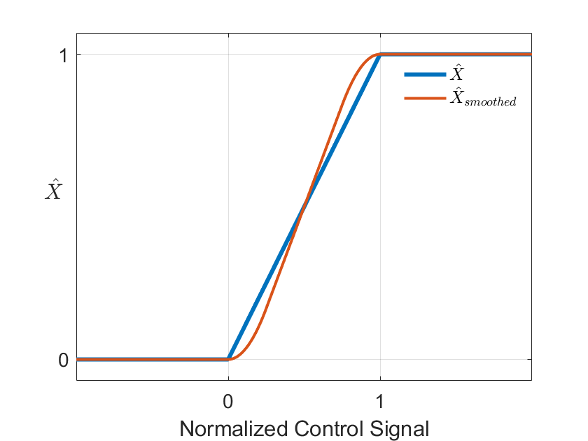## Numerical Smoothing

### Numerical Smoothing in Valve Opening Calculations

When a valve block that uses linear parameterization is in a near-open or near-closed position, variables such as valve area or control pressure transition from constant to linear regions. The transitions between the regions are sharp and their slopes discontinuous. These transitions pose a challenge to variable-step solvers and can extend the time needed to finish the simulation run. When you enable numerical smoothing, the block removes the discontinuities.

When the Smoothing factor parameter is nonzero, the block enables numerical smoothing. The block applies the smoothing to a normalized variable. For a variable X, the normalized variable, $\stackrel{^}{X}$, is

`$\stackrel{^}{X}=\frac{X-a}{b-a}$`

where a and b are the lower and upper limit of the variable, respectively. For most valve blocks, this limit depends on whether the valve is fully open or closed.

When you enable numerical smoothing, the block applies a smoothing factor, f, as

`${\stackrel{^}{X}}_{smoothed}=\frac{1}{2}+\frac{1}{2}\sqrt{{\stackrel{^}{X}}^{2}+{\left(\frac{f}{4}\right)}^{2}}-\frac{1}{2}\sqrt{{\left(\stackrel{^}{X}-1\right)}^{2}+{\left(\frac{f}{4}\right)}^{2}}.$`

The smoothing function impacts all calculated areas, but primarily influences the simulation at the extremes of the smoothed variable. This smoothing function applies to valves in the gas, thermal liquid, and isothermal liquid domains.

This image shows a discontinuous normalized variable, $\stackrel{^}{X}$, and a corresponding smoothed variable, ${\stackrel{^}{X}}_{smoothed}$, when the smoothing factor, f, is ``` 0.5```.After smoothing, the block reverts the variable to its original limits, `[`a,b`]`:

`${\text{X}}_{smoothed}={\stackrel{^}{X}}_{smoothed}\left(b-a\right)+a$`

A smoothing factor closer to `1` increases the effect of the smoothing function, while a smoothing factor closer to `0` decreases the effect.